## Posts

Showing posts with the label Pattern

### Print pattern in c - star pattern-5Print pattern in c - star pattern Write a program to print following pattern * ** *** **** Program code #include <stdio.h> int main () { int rows , i , j , space ; printf ( "Enter number of rows: " ); scanf ( "%d" ,& rows ); for ( i = rows ; i >= 1 ;-- i ) { for ( space = 0 ; space < rows - i ;++ space ) printf ( " " ); for ( j = i ; j <= 2 * i - 1 ;++ j ) printf ( "* " ); for ( j = 0 ; j < i - 1 ;++ j ) printf ( "* " ); printf ( "\n" ); } return 0 ; } You can Browse related article below for more information and program code related to recursive function call  Does above is helpful , Post you views in comment DO NOT MISS OTHER C PROGRAMMING TUTORIAL * indicates required Email Address *

### Print pattern in c - number pattern-11

Print pattern in c - number pattern Write a program to print following pattern 55555 4444 333 22 1 Program code #include <stdio.h> int main() {     int i, j;     for(i=5;i>=1;i--)     {         for(j=1;j<=i;j++)         {             printf("%d",i);         }         printf("\n");     }     return 0; } You can Browse related article below for more information and program code related to recursive function call  Does above is helpful , Post you views in comment DO NOT MISS OTHER C PROGRAMMING TUTORIAL * indicates required Email Address *

### Print pattern in c - star pattern-4

Print pattern in c - star pattern Write a program to print following pattern * * * * * * * * * * * * * * * * * * * * * * * * * Program code #include <stdio.h> int main () { int rows , i , j , space ; printf ( "Enter number of rows: " ); scanf ( "%d" ,& rows ); for ( i = rows ; i >= 1 ;-- i ) { for ( space = 0 ; space < rows - i ;++ space ) printf ( " " ); for ( j = i ; j <= 2 * i - 1 ;++ j ) printf ( "* " ); for ( j = 0 ; j < i - 1 ;++ j ) printf ( "* " ); printf ( "\n" ); } return 0 ; } You can Browse related article below for more information and program code related to recursive function call  Does above is helpful , Post you views in comment DO NOT MISS OTHER C PROGRAMMING TUTORIAL * indicates required Email Address *

### Print pattern in c - star pattern-3

Print pattern in c - star pattern Write a program to print following pattern * * * * * * * * * * * * * * * * * * * * * * * * * Program code #include <stdio.h> int main () { int i , space , rows , k = 0 ; printf ( "Enter the number of rows: " ); scanf ( "%d" ,& rows ); for ( i = 1 ; i <= rows ;++ i ) { for ( space = 1 ; space <= rows - i ;++ space ) { printf ( " " ); } while ( k != 2 * i - 1 ) { printf ( "* " ); ++ k ; } k = 0 ; printf ( "\n" ); } return 0 ; } You can Browse related article below for more information and program code related to recursive function call  Does above is helpful , Post you views in comment DO NOT MISS OTHER C PROGRAMMING TUTORIAL * indicates required Email Address *

### Print pattern in c - star pattern-2

Print pattern in c - star pattern Write a program to print following pattern * * * * * * * * * * * * * * * Program code #include <stdio.h> int main () { int i , j , rows ; printf ( "Enter the number of rows: " ); scanf ( "%d" ,& rows ); for ( i = rows ; i >= 1 ;-- i ) { for ( j = 1 ; j <= i ;++ j ) { printf ( "* " ); } printf ( "\n" ); } return 0 ; } You can Browse related article below for more information and program code related to recursive function call  Does above is helpful , Post you views in comment DO NOT MISS OTHER C PROGRAMMING TUTORIAL * indicates required Email Address *

### Print pattern in c - star pattern-1

Print pattern in c - star pattern Write a program to print following pattern * * * * * * * * * * * * * * * Program code #include <stdio.h> int main () { int i , j , rows ; printf ( "Enter the number of rows: " ); scanf ( "%d" ,& rows ); for ( i = 1 ; i <= rows ;++ i ) { for ( j = 1 ; j <= i ;++ j ) { printf ( "* " ); } printf ( "\n" ); } return 0 ; } You can Browse related article below for more information and program code related to recursive function call  Does above is helpful , Post you views in comment DO NOT MISS OTHER C PROGRAMMING TUTORIAL * indicates required Email Address *

### Print pattern in c - number pattern -10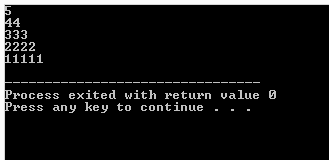Print pattern in c - number pattern Write a program to print following pattern 5 44 333 2222 11111 Program code #include <stdio.h> int main() { int i, j; for(i=5;i>=1;i--) { for(j=5;j>=i;j--) { printf("%d",i); } printf("\n"); } return 0; } Step by Step solution 1. Number of row -    Is has 5row  , so first loop will execute for 5 time #include <stdio.h> int main() {     int i, j;     for(i=5;i>=1;i--)     {...... }     return 0; } 2. Number of character -  It has only one type of character , so there will be only one nested loop #include <stdio.h> int main() { ...... for(j=5;j>=i;j--) { printf("%d",i); } printf("\n"); } 3.Number of column - It has 5 maximum column and is decreasing with row number , so the nested loop decrease it's repetation with increase in row #inc

### Print pattern in c - number pattern -9Print pattern in c - number pattern Write a program to print following pattern 1 22 333 4444 55555 Program code #include <stdio.h> int main() { int i, j; for(i=1;i<=5;i++) { for(j=1;j<=i;j++) { printf("%d",i); } printf("\n"); } return 0; } Step by Step solution 1. Number of row -    Is has 5row  , so first loop will execute for 5 time #include <stdio.h> int main() {     int i, j;     for(i=1;i<=5;i++)     {...... }     return 0; } 2. Number of character -  It has only one type of character , so there will be only one nested loop #include <stdio.h> int main() { ...... for(j=1;j<=i;j++) { printf("%d",i); } printf("\n"); } 3.Number of column - It has 5 maximum column and is decreasing with row number , so the nested loop decrease it's repetation with increase in row #i

### Print pattern in c - number pattern -8Print pattern in c - number pattern Write a program to print following pattern 5 54 543 5432 54321 Program code #include <stdio.h> int main() { int i, j; for(i=5;i>=1;i--) { for(j=5;j>=i;j--) { printf("%d",j); } printf("\n"); } return 0; } Step by Step solution 1. Number of row -    Is has 5row  , so first loop will execute for 5 time #include <stdio.h> int main() {     int i, j;     for(i=5;i>=1;i--)     {...... }     return 0; } 2. Number of character -  It has only one type of character , so there will be only one nested loop #include <stdio.h> int main() { ...... for(j=5;j>=i;j--) { printf("%d",j); } printf("\n"); } 3.Number of column - It has 5 maximum column and is decreasing with row number , so the nested loop decrease it's repetation with increase in row #i

### Print pattern in c - number pattern-7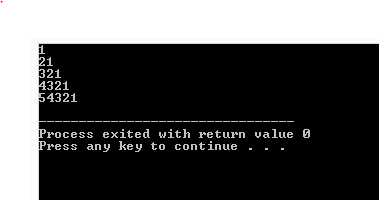Print pattern in c - number pattern Write a program to print following pattern 1 21 321 4321 54321 Program code #include <stdio.h> int main() { int i, j; for(i=1;i<=5;i++) { for(j=i;j>=1;j--) { printf("%d",j); } printf("\n"); } return 0; } Step by Step solution 1. Number of row -    Is has 5row  , so first loop will execute for 5 time #include <stdio.h> int main() {     int i, j;     for(i=1;i<=5;i++)     {...... }     return 0; } 2. Number of character -  It has only one type of character , so there will be only one nested loop #include <stdio.h> int main() { ...... for(j=i;j>=1;j--) { printf("%d",j); } printf("\n"); } 3.Number of column - It has 5 maximum column and is decreasing with row number , so the nested loop decrease it's repetation with increase in row #i

### Print pattern in c - number pattern -6Print pattern in c - number pattern -6 Write a program to print following pattern 5 45 345 2345 12345 Program code #include <stdio.h> int main() { int i, j; for(i=5;i>=1;i--) { for(j=i;j<=5;j++) { printf("%d",j); } printf("\n"); } return 0; } Step by Step solution 1. Number of row -    Is has 5row  , so first loop will execute for 5 time #include <stdio.h> int main() {     int i, j;     for(i=5;i>=1;i--)     {...... }     return 0; } 2. Number of character -  It has only one type of character , so there will be only one nested loop #include <stdio.h> int main() { ...... for(j=i;j<=5;j++) { printf("%d",j); } printf("\n"); } 3.Number of column - It has 5 maximum column and is decreasing with row number , so the nested loop decrease it's repetation with increase in row #i

### Print pattern in c - number pattern -5Print pattern in c - number pattern -5 Write a program to print following pattern 1 12 123 1234 12345 Program code #include <stdio.h> int main() { int i, j; for(i=1;i<=5;i++) { for(j=1;j<=i;j++) { printf("%d",j); } printf("\n"); } return 0; } Step by Step solution 1. Number of row -    Is has 5row  , so first loop will execute for 5 time #include <stdio.h> int main() {     int i, j;     for(i=1;i<=5;i++)     {...... }     return 0; } 2. Number of character -  It has only one type of character , so there will be only one nested loop #include <stdio.h> int main() { ...... for(j=1;j<=i;j++) { printf("%d",j); } printf("\n"); } 3.Number of column - It has 5 maximum column and is decreasing with row number , so the nested loop decrease it's repetation with increase in row

### Print pattern in c - number pattern -4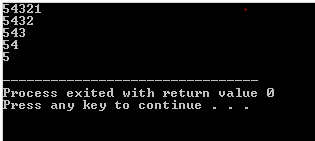Print pattern in c - number pattern -4 Write a program to print following pattern 54321 5432 543 54 5 Program code #include <stdio.h> int main() { int i, j; for(i=1;i<=5;i++) { for(j=5;j>=i;j--) { printf("%d",j); } printf("\n"); } return 0; } Step by Step solution 1. Number of row -    Is has 5row  , so first loop will execute for 5 time #include <stdio.h> int main() {     int i, j;     for(i=1;i<=5;i++)     {...... }     return 0; } 2. Number of character -  It has only one type of character , so there will be only one nested loop #include <stdio.h> int main() { ...... for(j=5;j>=i;j--) { printf("%d",j); } printf("\n"); return 0; } 3.Number of column - It has 6 maximum column and is decreasing with row number , so the nested loop decrease it's repetation with

### Print pattern in c - number pattern -3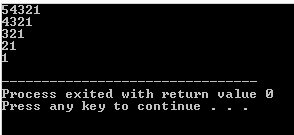Print pattern in c - number pattern -3 Write a program to print following pattern 54321 4321 321 21 1 Program code #include <stdio.h> int main() { int i, j; for(i=5;i>=1;i--) { for(j=i;j>=1;j--) { printf("%d",j); } printf("\n"); } return 0; } Step by Step solution 1. Number of row -    Is has 5row  , so first loop will execute for 5 time #include <stdio.h> int main() {     int i, j;     for(i=5;i>=1;i--)     {...... }     return 0; } 2. Number of character -  It has only one type of character , so there will be only one nested loop #include <stdio.h> int main() { ...... for(j=i;j>=1;j--) { printf("%d",j); } printf("\n"); } 3.Number of column - It has 5 maximum column and is decreasing with row number , so the nested loop decrease it's repetation with increase in row #include <stdio.h>

### Print pattern in c - number pattern -2

Print pattern in c - number pattern -2 Write a program to print following pattern 12345 2345 345 45 5 Program code #include <stdio.h> int main() {     int i, j;     for(i=1;i<=5;i++)     {         for(j=i;j<=5;j++)         {             printf("%d",j);         }         printf("\n");     }     return 0; } Step by Step solution 1. Number of row -    Is has 5row  , so first loop will execute for 5 time #include <stdio.h> int main() {     int i, j;     for(i=1;i>=5;i++)     {...... }     return 0; } 2. Number of character -  It has only one type of character , so there will be only one nested loop #include <stdio.h> int main() { ...... for(j=i;j<=5;j++)         {             printf("%d",j);         }         printf("\n"); return 0; } 3.Number of column - It has 6 maximum column and is decreasing with row number , so the nested loop decrease it's repetation with increase

### print pattern in c - number pattern -1

Print pattern in c - number pattern -1 Write a program to print following pattern 123456 12345 1234 123 12 1 Program code #include <stdio.h> int main() {     int i, j; for(i=6;i>=1;i--) {         for(j=1;j<=i;j++)         {             printf("%d",j);         }         printf("\n");     }     return 0; } Step by Step solution 1. Number of row -    Is has 6 row  , so first loop will execute for 6 time #include <stdio.h> int main() {     int i, j;     for(i=6;i>=1;i--)     {...... }     return 0; } 2. Number of character -  It has only one type of character , so there will be only one nested loop #include <stdio.h> int main() { ...... for(j=1;j<=i;j++)         {             printf("%d",j);         } return 0; } 3.Number of column - It has 6 maximum column and is decreasing with row number , so the nested loop decrease it's repetation with increase in row #include <std

### Print pattern in c - explore new perspectives in pattern printing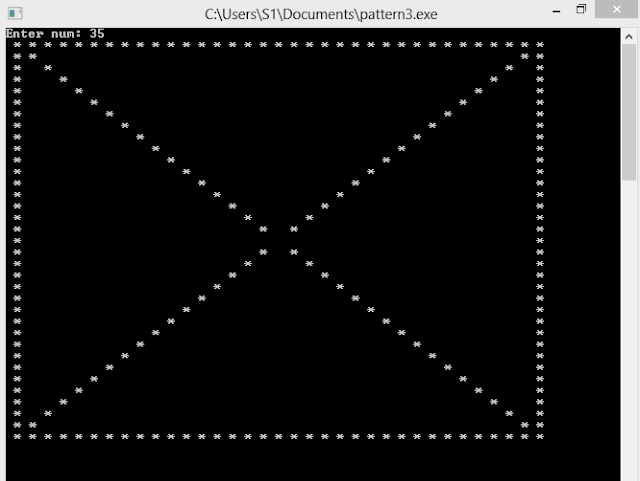Print pattern in C You will be able to : >> Design any kind of pattern >>Understand element of pattern designing >>Understand patterns >>Understand loop in c >>Understand Sequence analysis What is Pattern? A pattern is set of  same or different kind of things that represent definite geometrical shapes or size. Geometrical shape can be  Triangle Square Pramid If you look above picture than difinetly you will find repetition of shapes or color in definite manner .  It's example of pattern. For Creating sucessfull Pattern , You must know Loop Element of pattern Sequence analysis of pattern Loop loop are the control statement . They are used when programmer needs to execute certain part of program for definite repetation Different kinds of loop are For loop While loop Do while loop Among these for loop is used when the number of repetition is known. Although while and do-while loop can be used while n